International Journal of Information and Communication Sciences
Volume 1, Issue 3, December 2016, Pages: 47-53

Compact Rectangular Slot Patch Antenna for Dual Frequency Operation Using Inset Feed Technique

Jeffrey C. Saturday, Kufre M. Udofi, Akaninyene B. Obot

Department of Electrical/Electronic and Computer Engineering, University of Uyo, Uyo, Nigeria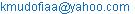(K. M. Udofia)

Jeffrey C. Saturday, Kufre. M. Udofi, Akaninyene. B. Obot. Compact Rectangular Slot Patch Antenna for Dual Frequency Operation Using Inset Feed Technique. International Journal of Information and Communication Sciences. Vol. 1, No. 3, 2016, pp. 47-53. doi: 10.11648/j.ijics.20160103.13

Received: October 16, 2016; Accepted: December 28, 2016; Published: January 14, 2017

Abstract: In recent years, the inventions in communication systems require the development of low cost, minimal weight, compact and low profile antennas that are capable of maintaining high performance over a wide spectrum of frequencies. This technological trend has focused much effort in the design of microstrip patch antennas because of its inherent characteristics that fits the requirements of modern communication devices. This paper presents the design and simulation of a compact rectangular slot patch antenna for dual frequency operation at 2.4 GHz and 5.2 GHz using the inset feed technique. The simulation of the designed antenna was done with the aid of computer simulation technology (CST) microwave studio version 12. The antenna has a miniature compact size of 44×41×1.6 mm3 which resonated at a return loss of -19.619 dB and voltage standing wave ratio (VSWR) of 1.303 at 2.4 GHz, and -17.55 dB and VSWR of 1.301 at 5.2 GHz. The results show that the antenna has a corresponding bandwidth of 124.6 MHz at 2.4 GHz and 119.8 MHz at 5.2 GHz. The substrate used in the proposed antenna is the flame resistant four (FR-4) with a dielectric constant of 4.4 and a loss tangent of 0.023. The proposed antenna may find applications in wireless local area network (WLAN) and Bluetooth technology.

Keywords: Antenna, Slot Patch, Dual Frequency, Compact, Inset Feed

Contents

1. Introduction

The inventions of new wireless communication systems have increased the demand for antennas capable of being embedded in portable devices which serve as WLAN, mobile or terrestrial-satellite transceivers. With time and increased demand for sophistication, electronic devices have become smaller in size and hence antennas required for radiating or receiving signals have also become smaller and lightweight [1, 2]. As a matter of fact, microstrip antennas (MSAs) can meet these requirements as they are lightweight and have low profile, it is feasible for them to be structured conformably to the mounting hosts. Moreover, they are easy to fabricate, have low cost and are easily integrated into arrays or into microwave printed circuits for bandwidth augmentation .

The rectangular microstrip antenna consists of a rectangular metallic radiation patch of size Lp × Wp incorporated to one side of a dielectric substrate of size Lg × Wg with relative permittivity Ɛr and thickness h, which has a metallic ground plane on the other side . The radiating patch, which can take any possible shape as well as feeding network, implementable with different technique are usually photo-etched on the dielectric substrate.

Dual band operation can be realized from a single feed using slot loaded or stacked microstrip antenna or two separately fed antennas sharing a common aperture . The former design, when used in arrays, has certain limitations like complicated beam forming or duplexing network and difficulty in realizing good radiation patterns at both bands. The second technique provides more flexibility with separate feed system as beams in each frequency band can be controlled independently. Another desirable feature of a dual band antenna is easy adjustability of upper and lower frequency bands.

2. Review of Related Works

Several techniques have been applied to overcome the problem of narrow bandwidth which is an inherent drawback in using microstrip patch antennas in a variety of application; these techniques include increasing the substrate thickness, introducing parasitic elements (co-planar or stack configuration), or modifying the patch’s shape itself . Modifying patch’s shape includes designing a rectangular shaped slot on the radiating patch antennas. A lot of papers have been published highlighting different techniques of using dual frequency operation to enhance bandwidth [3-9] but the method proposed in this paper gives more versatility of mounting the antenna both on planar and non-planar surfaces aside using for mobile devices.

Use of multiple patches was suggested by  but the ripples as observed from the return loss plot when used in practical application can interfere with adjacent frequencies. Slotted square patch with inset feed was proposed by , it was supposed to cover 2.4 GHz, 5.2 GHz and 5.8 GHz respectively but it fell short as it only managed to cover only 2.6 GHz and 5.2 GHz from the return loss plot obtained. Another Square slotted patch with quarter-wave edge feed was proposed by  which resonated at 2.5 GHz and 5 GHz as designed, though it resonated at the designed frequency but the frequency covered differ from the one proposed in this pater.

3. Antenna Design Procedure

For design, the procedure starts with the calculation of the length and width of conventional single band microstrip patch antenna using standard antenna design equations from [2, 10] at 2.4 GHz. The first design step is to choose a suitable dielectric substrate of appropriate thickness (h). A flame resistant substrate with dielectric constant Ɛr =4.4 having thickness (h) =1.6 mm is chosen as the substrate material for the patch antenna. Furthermore, the dimensions of the patch and feed line are determined and the feed line is placed properly to resonate at 2.4 GHz. Then the modifications of the antenna structure are carried out to create dual resonance. For efficient radiation, patch parameters are determined as illustrated in the following steps;

Step One: The resonant frequency (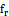), select substrate relative permittivity (Ɛr) and a substrate thickness (h) are specified. The loss due to surface waves can be neglected when h satisfies the criterion in Equation (1) according to  given as:

h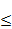0.3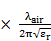(1)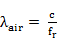(2)

Where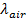= wavelength in free space (air), c = speed of light = 3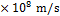,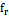= the selected resonant frequency = 2.4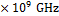, loss tangent (tan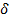) = 0.023

Step Two: Calculate the width of the patch Wp thus;

Wp =(3)

Step Three: The effective dielectric constantis determined based on Equation (4);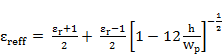For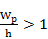(4)

Step Four: calculate the normalized extension length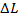given as;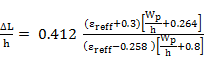(5)

Step Five: The value of the length of the patch Lp is given by,

L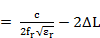p (6)

Step Six: Calculate the notch width, g using the equation from  thus:

g =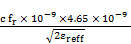(7)

Step Seven: Compute the resonant input resistance Rin thus;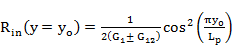(8)

The equation for the characteristic impedance Zo is given by  as;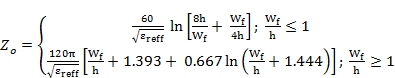(9)

In this design, the second expression in Equation 9 applies. Hence;

Rin(edge) =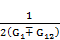(10)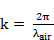(11)

I1 = -2 + cos(X) + XSi(X) +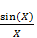(12)

X = kWp (13)

G1 =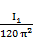(14)

G12 =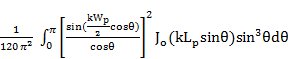(15)

Where Jo is the Bessel function of the first kind of order zero, G1 is conductance of microstrip radiator and G12 is the mutual conductance. For this design, input impedance of 50 Ω was used.

Step Eight: Calculate the inset feed recessed distance y0 and the width of the transmission line Wf using the equation for resonant input impedance thus;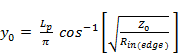(16)

According to , the width of the transmission line is calculated thus; for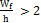;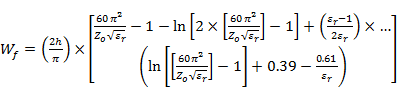(17)

Step Nine: Calculate the ground plane dimensions thus; the length (Lg) and width (Wg) of the ground plane is:

Lg = 6h + Lp (18)

(Lg) = 6h + Wg (19)

Other parameters such as A, L1, L2, W1, W2, C, b, n, m were calculated using equations from .

The summary of the results of the design equations is shown in Table 1.

Table 1. Design values of the proposed dual band antenna after optimization.

 Variables Wg Lg Wp Lp Wf Lf g yo C b A L1 W1 L2 W2 n m Value (mm) 44 41 24 21 2.96 10 2 5.2 10 13 2.3 20 4.5 22 12.3 12 26

The geometry of the proposed inset fed dual-band antenna is shown in Figure 1 while the design of the dual-band antenna in CST Microwave Studio [14, 15] is shown in Figure 2.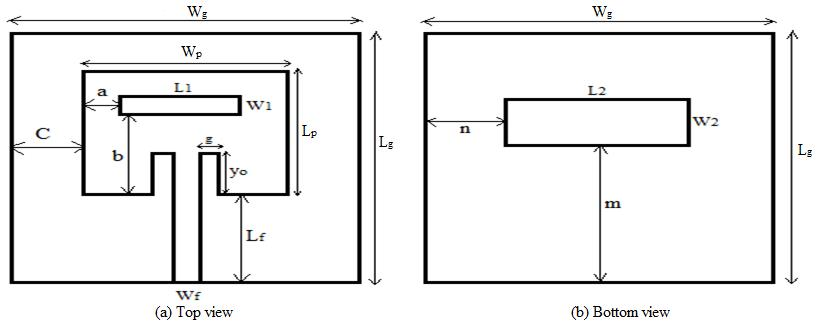Figure 1. Geometry of the proposed dual-band antenna.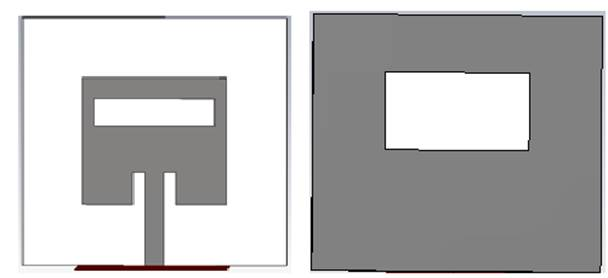Figure 2. Designed dual-band antenna in CST Microwave Studio.

4. Results and Discussion

The simulation results using CST Microwave Studio are as shown in Figure 3 to Figure 9.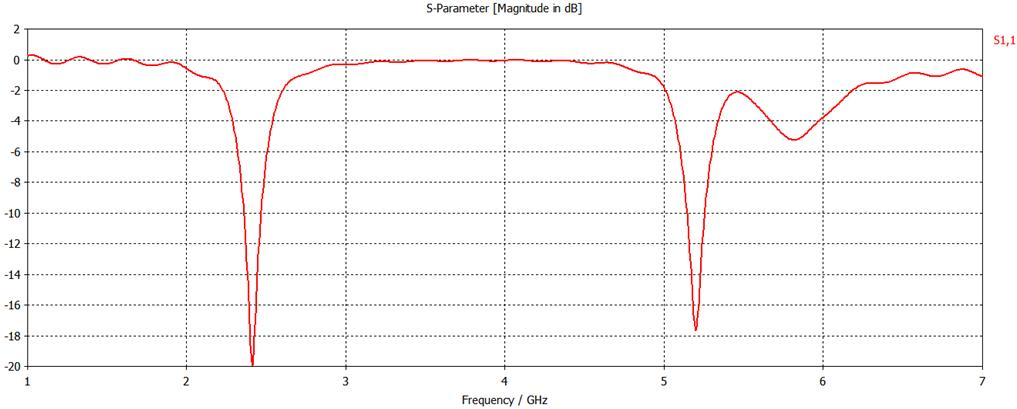Figure 3. Return loss plot of the proposed antenna.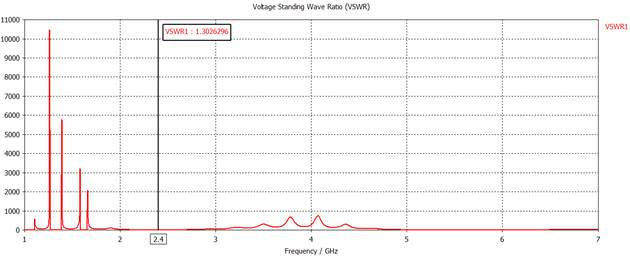Figure 4. Voltage Standing Wave Ratio (VSWR) of the proposed antenna at 2.4 GHz.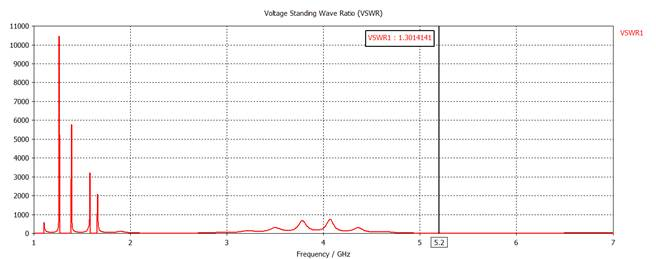Figure 5. Voltage Standing Wave Ratio (VSWR) of the proposed antenna at 5.2 GHz.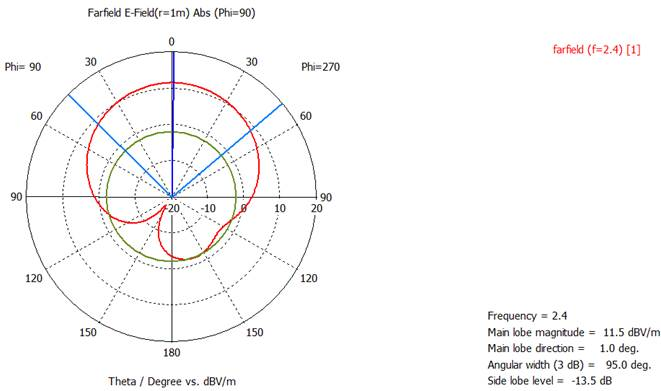Figure 6. E-Plane Radiation pattern at 2.4 GHz.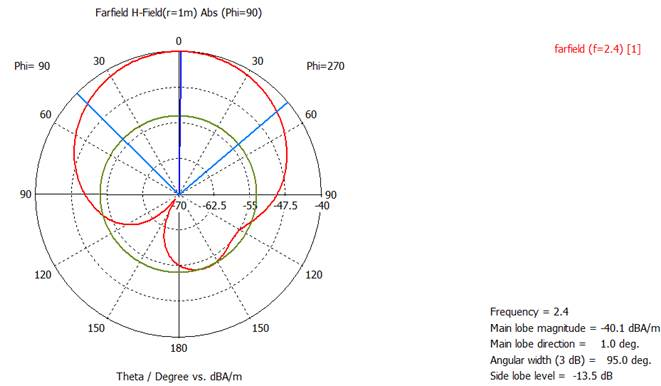Figure 7. H-Plane Radiation pattern at 2.4 GHz.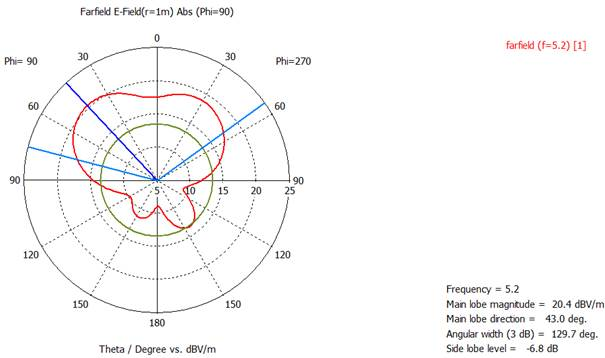Figure 8. E-Plane Radiation pattern of the proposed antenna at 5.2 GHz.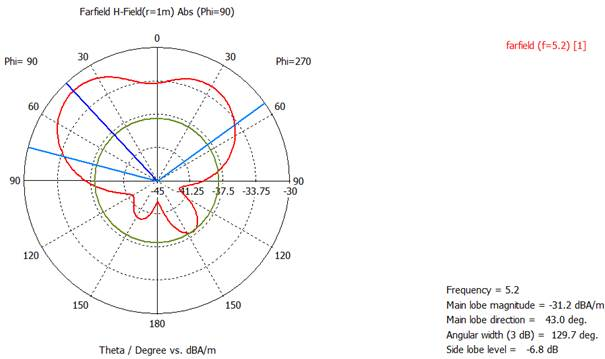Figure 9. H-Plane Radiation pattern of the proposed antenna at 5.2 GHz.

The return loss of the proposed antenna as can be seen from Figure 3 shows that at -19.619 dB, the resonance frequency is 2.4189 GHz and at -17.55 dB the resonance frequency is 5.2034 GHz. The 124.6 MHz at 2.4 GHz and 119.8 MHz at 5.2 GHz, the respective bandwidths of the antenna can be calculated according to  thus;

Bandwidth at 2.4 GHz == 5.4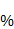Bandwidth at 5.2 GHz =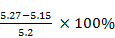= 2.3Figures 4 and Figure 5 show the VSWR of the proposed antenna indicating that they resonated within the allowed specification for good design (1 ≤ VSWR ≤ 2). It is seen from Figure 4 that VSWR of 1.303 at 2.4 GHz was achieved while a VSWR of 1.301 at 5.2 GHz was achieved.

The radiation pattern of the dual band antenna at 2.4 GHz and 5.2 GHz is shown in Figure 6 to Figure 9. From Figures 6 and Figure 7, the half power beam width (HPBW) of the antenna at 2.4 GHz is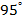, main lobe magnitude is 11.5 dBV/m in the E-field and -40.1 dBA/m in the H-field, sidelobe level is at -13.5 dB and the main lobe direction is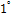. At 5.2 GHz as seen from Figure 8 and Figure 9, the HPBW of the antenna is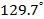, main lobe magnitude is 20.4 dBV/m in the E-field and -31.2 dBA/m in the H-field, side lobe level is at -6.8 dB and the main direction is at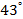.

5. Conclusion

In this paper, dual band antenna has been designed and simulated, observations have been made on several parameters such as return loss, radiation pattern and VSWR in both cases. From the results of the simulation, it has been observed that the parameters influencing the antenna are the relative permittivity of the dielectric material under the patch, the width (Wf) of the microstrip line, the position of the slot on the patch and the ground plane (in case of the dual band), and the length and width of the patch. With a BW of 115 MHz which represents 5.4% at 2.4 GHz and 110 MHz representing a BW of 2.3% at 5.2 GHz, a VSWR of 1.303 at 2.4 GHz and 1.301 at 5.2 GHz the designed antenna has met the objectives outlined.

References

1. Balanis, C.A., Antenna theory: analysis and design. 2016: John Wiley & Sons.
2. Constantine, A.B., Antenna theory: analysis and design. Microstrip Antennas, third edition, John wiley & sons. 2005.
3. Garg, R., Microstrip antenna design handbook. 2001: Artech house.
4. Hamad, E.K., Design and Implementation of Dual-Band Microstrip Antennas for Rfid Reader Application. Cienca E Tec. Vitivinic, 2014. 29 (9): p. 1-10.
5. Jain, K. and S. Sharma, Dual Band Rectangular Microstrip Antenna for Wireless Communication Systems. International journal of Innovations in Engineering and Techonology (IJIET), 2013. 2 (4): p. 235-246.
6. Asrokin, A., M. Rahim, and M.A. Aziz. Dual band microstrip antenna for wireless LAN application. in Applied Electromagnetics, 2005. APACE 2005. Asia-Pacific Conference on. 2005. IEEE.
7. Nag, V.R. and G. Singh, Design and Analysis of Dual Band Microstrip Patch Antenna with Microstrip feed line and slot for Multiband Application in Wireless Communication. IRACST - International Journal of Computer Science and Information Technology & Security (IJCSITS), 2012. 2 (6): p. 1266-1270.
8. Mansour, Y.E., Single slot dual band microstrip antenna for WiMAX application. Atilim University, June, 2014.
9. Lu, J.-H., Single-feed dual-frequency rectangular microstrip antenna with pair of step-slots. Electronics letters, 1999. 35 (5): p. 354-355.
10. Kumar, G. and K. Ray, Broadband microstrip antennas. 2002: Artech House.
11. Pozar, D.M., Microwave engineering. 2009: John Wiley & Sons.
12. Ali, M., et al. A design of reconfigurable rectangular microstrip slot patch antennas. in System Engineering and Technology (ICSET), 2011 IEEE International Conference on. 2011. IEEE.
13. Shafai, L., Wideband Microstrip Antennas. Antenna engineering handbook, 4th ed., McGraw-Hill Professional, New York, 2007: p. 574-623.
14. Studio, C.M., 3-D Electromagnetic Simulation Software. CST Corp., Darmstadt, 2015.
15. Studio, M., CST-Computer Simulation Technology. Bad Nuheimer Str, 2008. 19: p. 64289.
16. Siakavara, K., Methods to design microstrip antennas for modern applications. 2011: INTECH Open Access Publisher.

 Contents 1. 2. 3. 4. 5.
Article ToolsAbstractPDF(2979K)